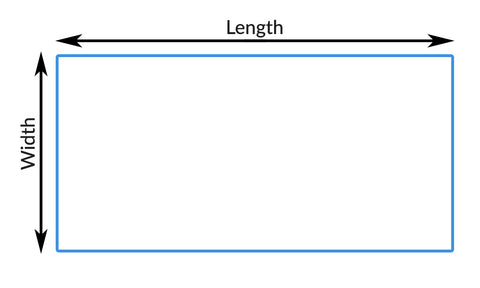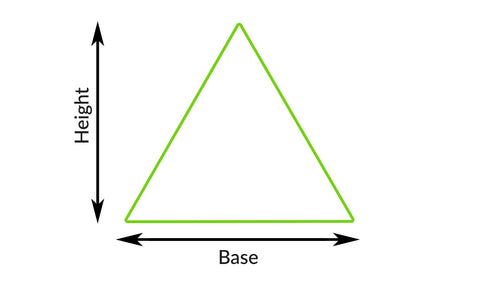# How to measure a floor

To calculate how much square foot or meters you'll need for vinyl floor installation, you'll need to know the total area.

For Squares and RectanglesFor square and rectangle areas, the formula would be:

length * width = area

So if a rectangle's length is 15 and its width is 10:

15 * 10 = 150 square feet / meters needed

Usually, you want to add the "waste factor," which accounts for any damages or extra cuts you'll need. The standard waste number is 10%.

With waste, the final formula will now be:

area * (1 + waste number) = square feet / meters needed

Using the area (150) calculated above, this would be:

150 * (1 + 0.1)

OR

150 * 1.1 = 165 square feet / meters needed

For TrianglesTo calculate triangles, the formula will be:

1/2 (base) * height = area

So if the base is 12 and the height is 9, then we'll get:

1/2 (12) * 9 = area

OR

6 * 9 = 54 square feet / meters

With the waste factor, the final formula is the same as with squares and rectangles:

area * (1 + waste number) = square feet / meters needed

So using the triangle's area (54) above and a waste of 10%, we get:

54 * (1 + 0.1) = square feet / meters needed

OR

54 * 1.1 = 59.4 square feet / meters needed

For rooms with both Triangle and Square/Rectangle shaped roomsFor rooms that have a both a triangle and square/rectangle shape, you'll have to calculate each area separately. Use the square/rectangle and triangle area formulas (with waste included) above.